• c程序设计第五版谭浩强课后习题答案 第一章 1.什么是程序?什么是程序设计? 程序：就是一组能识别和执行的指令，每一条指令使计算机执行特定的操作 程序设计：是指从确定任务到得到结果、写出文档的全过程 2.为什么...
c程序设计第五版谭浩强课后习题答案
第一章
1.什么是程序?什么是程序设计?
程序：就是一组能识别和执行的指令，每一条指令使计算机执行特定的操作
程序设计：是指从确定任务到得到结果、写出文档的全过程
2.为什么需要计算机语言?高级语言有哪些特点?
为什么需要计算机语言：计算机语言解决了人和计算机交流是的语言问题，使得计算机和人都能识别
高级语言有哪些特点：
​	2.1 高级语言的数据结构要比汇编和机器语言丰富；
​	2.2 高级语言与具体机器结构的关联没有汇编以及机器语言密切；
​	2.3 高级语言更接近自然语言更容易掌握；
​	2.4 高级语言编写的程序要经过编译或解释计算机才能执行;
3.正确理解以下名词及其含义:
(1)源程序,目标程序,可执行程序。
​	源程序：指未编译的按照一定的程序设计语言规范书写的文本文件，是一系列人类可读的计算机语言指令
​	目标程序：为源程序经编译可直接被计算机运行的机器码集合，在计算机文件上以.obj作扩展名
​	可执行程序：将所有编译后得到的目标模块连接装配起来，在与函数库相连接成为一个整体，生成一个可供计算机执行的目标程序，成为可执行程序
(2)程序编辑,程序编译,程序连接。
程序编辑：上机输入或者编辑源程序。
程序编译：

先用C提供的“预处理器”，对程序中的预处理指令进行编译预处理
对源程序进行语法检查， 判断是否有语法错误，直到没有语法错误未知
编译程序自动把源程序转换为二进制形式的目标程序

程序连接：将所有编译后得到的目标模块连接装配起来，在与函数库相连接成为一个整体的过程称之为程序连接
(3)程序,程序模块,程序文件。
程序：一组计算机能识别和执行的指令，运行于电子计算机上，满足人们某种需求的信息化工具
程序模块：可由汇编程序、编译程序、装入程序或翻译程序作为一个整体来处理的一级独立的、可识别的程序指令
程序文件：程序的文件称为程序文件，程序文件存储的是程序，包括源程序和可执行程序
(4)函数,主函数，被调用函数,库函数。
函数：将一段经常需要使用的代码封装起来，在需要使用时可以直接调用，来完成一定功能
主函数：又称main函数，是程序执行的起点
被调用函数：由一个函数调用另一个函数，则称第二个函数为被调用函数
库函数：一般是指编译器提供的可在c源程序中调用的函数。可分为两类，一类是c语言标准规定的库函数，一类是
​		编译器特定的库函数
(5)程序调试,程序测试。
程序调试：是将编制的程序投入实际运行前，用手工或编译程序等方法进行测试，修正语法错误和逻辑错误的过程
程序测试：是指对一个完成了全部或部分功能、模块的计算机程序在正式使用前的检测，以确保该程序能按预定的
​	 	   方式正确地运行
4.编写一个C程序,运行时输出
Hello World!
这个程序是一些国外C教材中作为第一个程序例子介绍的，一般称为Hello程序。
代码示例：
#include <stdio.h>

int main()
{
printf("%s\n", "Hello World!");
return 0;
}

运行截图：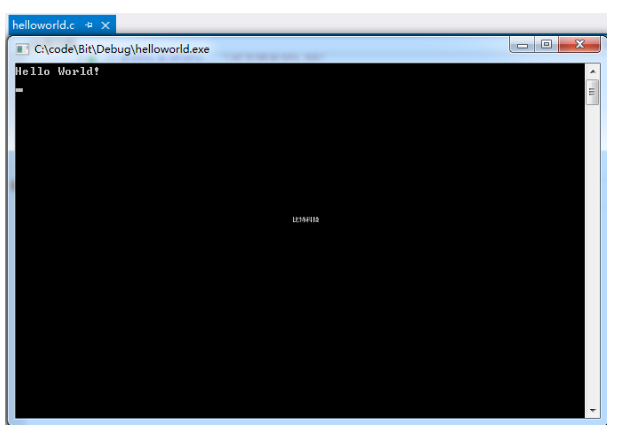5.编写一个C程序,运行时输出以下图形:
****
****
​    ****
​      ****
代码示例：
#include <stdio.h>

int main()
{
for (int i = 0; i < 4; i++)
{
for (int j = 0; j < i; j++)
{
printf("%s", "  ");
}
printf("%s\n", "*****");
}
return 0;
}

运行截图：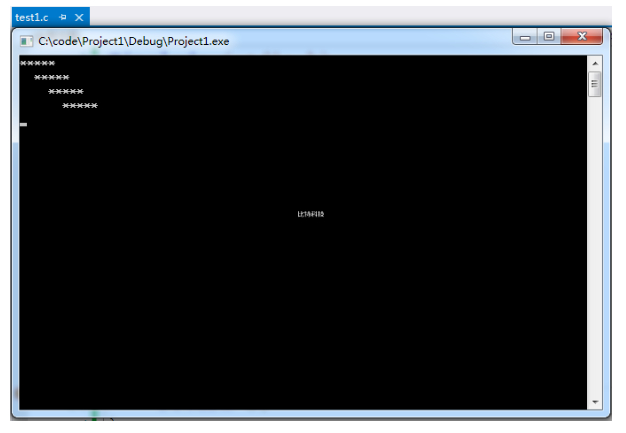c程序设计
6.编写一个C程序,运行时输人a,b,c三个值,输出其中值最大者。
代码示例：
#include<stdio.h>

int main()
{
int a, b, c, max;
printf("请输入三个数：\n");

scanf("%d%d%d", &a, &b, &c);
if (a > b)
{
max = a;
}
else
{
max = b;
}

if (c > max)
{
max = c;
}
printf("三个数中最大的数为：%d", max);
return 0;

}

运行截图：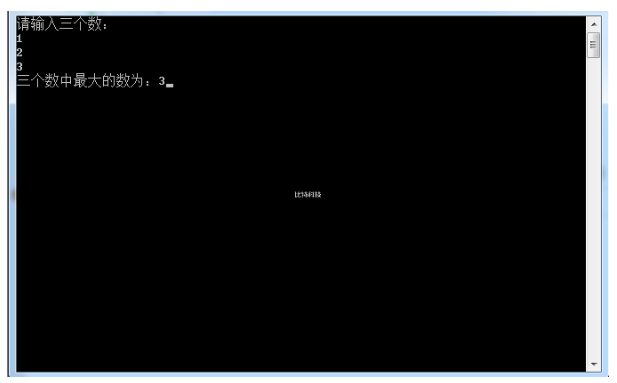7.看懂《C程序设计(第五版)学习辅导》第16章中介绍的用Visual Studio 2010对C程序进行编辑、编译、连接和运行的方法,并进行以下操作:
(1)建立一个新项目,定名为project1.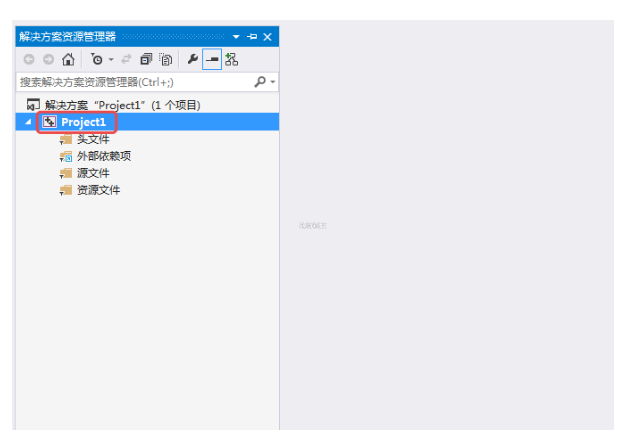(2)建立一个新文件,定名为test1。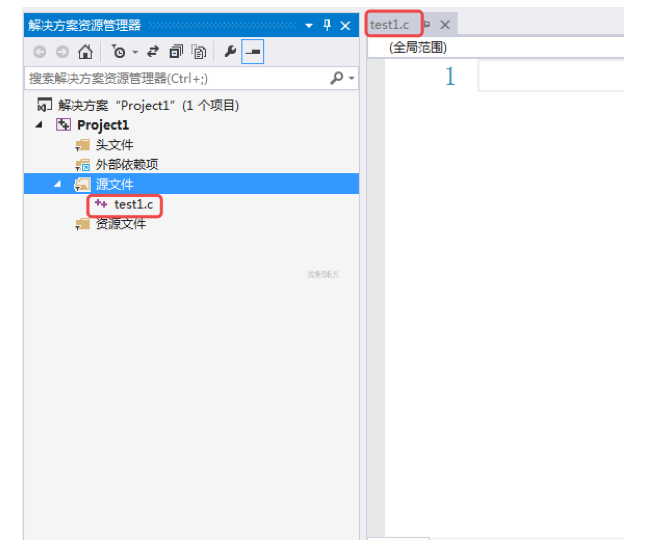(3)向test1 文件输人源程序(此源程序为读者自己编写的程序)。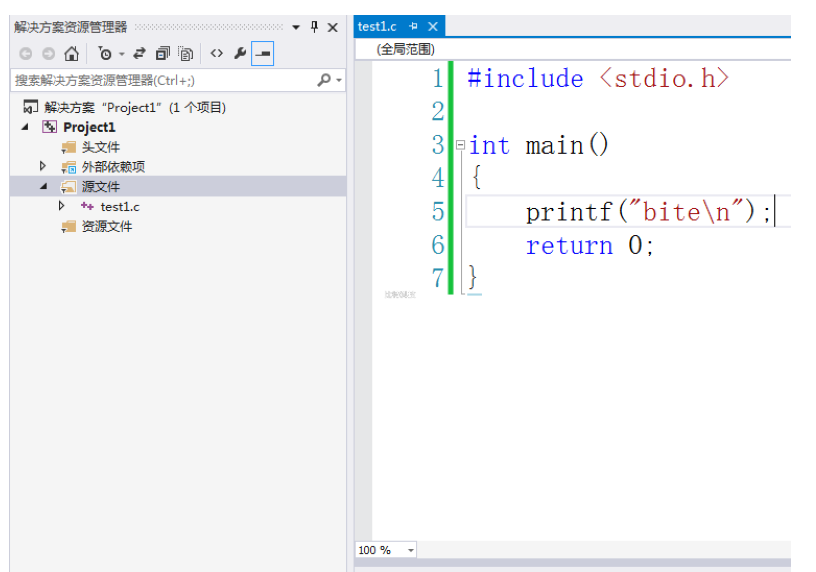(4) 编译该源程序,如发现程序有错，请修改之,直到不出现“编译出错”为止。
(5) 连接并运行, 得到结果。分析结果。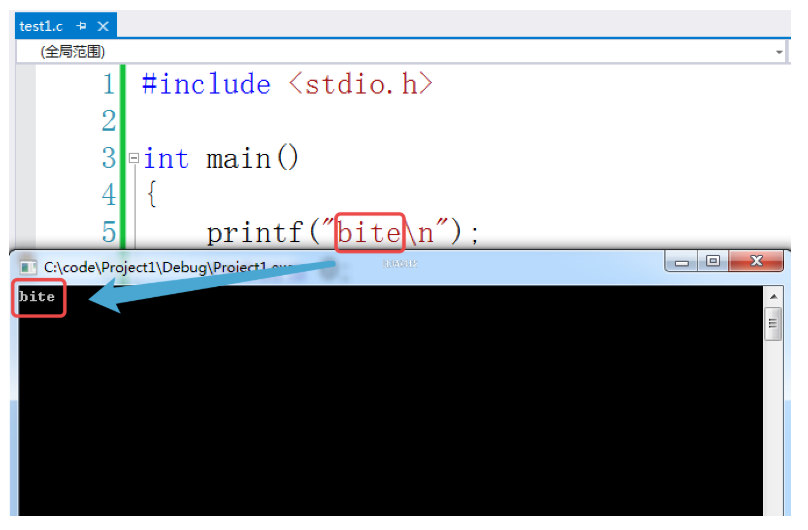展开全文c程序设计
• C语言程序设计第五版谭浩强课后答案 这里写目录标题C语言程序设计第五版谭浩强课后答案第八章 善于利用指针1. 输入3个整数，要求按由小到大的顺序输出。2. 输入3个字符串，要求按由小到大的顺序输出。3. 输入10个...
C语言程序设计第五版谭浩强课后答案
C语言程序设计第五版第八章答案C语言程序设计第五版谭浩强课后答案第八章 善于利用指针1. 输入3个整数，要求按由小到大的顺序输出。2. 输入3个字符串，要求按由小到大的顺序输出。3. 输入10个整数,将其中最小的数与第一个数对换, 把最大的数与最后一个数对换。①输人10个数;②进行处理;③输出10个数。4. 有n个整数,使前面各数顺序向后移m个位置,最后m个数变成最前面m个数,见图8.43。 写一函数实现以上功能,在主函数中输人n个整数和输出调整后的n个数。5. 有n个人围成一圈，顺序排号。从第1个人开始报数(从1到3报数),凡报到3的人退出圈子,问最后留下的是原来第几号的那位。6. 写一函数,求一个字符串的长度。在main函数中输入字符串,并输出其长度。7. 有一字符串,包含n个字符。写一函数,将此字符串中从第m个字符开始的全部字符复制成为另一个字符串。8. 输入一行文字,找出其中大写字母、小写字母、空格、数字以及其他字符各有多少。9. 写一函数,将一个3x3的整型矩阵转置。10. 将一个5x5的矩阵中最大的元素放在中心,4个角分别放4个最小的元素(顺序为从左到右,从上到下依次从小到大存放),写一函数实现之。用main函数调用。11. 在主函数中输入10个等长的字符串。用另一函数对它们排序。然后在主函数输出这10个已排好序的字符串。12. 用指针数组处理上一题目,字符串不等长。13. 写一个用矩形法求定积分的通用函数,分别求$\int_0^1 sinxdx, \quad \int_0^1 cosxdx, \quad \int_0^1 e^xdx,$ ,说明: sin,cos,exp 函数已在系统的数学函数库中，程序开头要用#include 第八章 善于利用指针
本章习题均要求使用指针方法处理。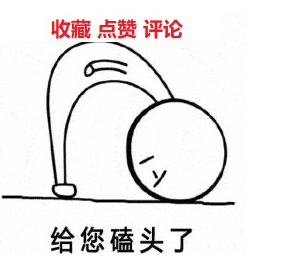1. 输入3个整数，要求按由小到大的顺序输出。
解题思路： 先获取到三个变量的地址，然后获取三个数据，通过指针进行比较转换即可
答案：
#include <stdio.h>

void swap(int *p_a, int *p_b)
{
int temp = *p_a;
*p_a = *p_b;
*p_b = temp;
}
int main()
{
int a, b, c, *p_a = &a, *p_b = &b, *p_c = &c;	 // 获取每个变量空间的地址
printf("Please enter three numbers:");
scanf_s("%d%d%d", p_a, p_b, p_c);
if (*p_a > *p_b) {
swap(p_a, p_b);//通过指针进行指向空间内的数据交换
}
if (*p_a > *p_c) {
swap(p_a, p_c);
}
if (*p_b > *p_c) {
swap(p_b, p_c);
}
printf("%d %d %d\n", *p_a, *p_b, *p_c);
system("pause");
return 0;
}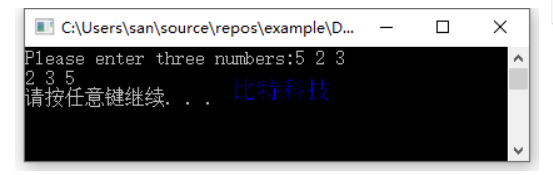2. 输入3个字符串，要求按由小到大的顺序输出。
解题思路： 字符串的比较可以使用strcmp函数，返回值>0表示大于，返回值小于0表示小于，返回追等于0表示相同。其他的比较排序思路与数字的排序交换没有区别，逐个进行比较先找出最大的，然后找出第二大的。
答案：
#include <stdio.h>
int main()
{
char str;
char *p;
printf("Please enter three strings:");
for (int i = 0; i < 3; i++) {
p[i] = str[i];
scanf_s("%s", p[i], 32);//后边的数字限制缓冲区边界，防止缓冲区溢出访问越界
}
//让p和p/p分别进行比较，找出最大的字符串，i+1之后，则让p和p进行比较，找出第二大
//i循环总个数-1次,最后一个是不需要比较的
for (int i = 0; i < 2; i++) {
for (int j = i + 1; j < 3; j++) {
if (strcmp(p[i], p[j]) > 0) {
char *tmp = p[i]; p[i] = p[j]; p[j] = tmp;
}
}

}
printf("%s %s %s\n", p, p, p);
system("pause");
return 0;
}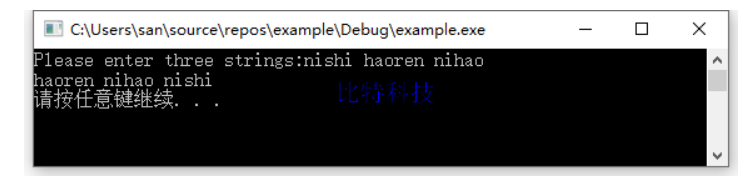3. 输入10个整数,将其中最小的数与第一个数对换, 把最大的数与最后一个数对换。
写3个函数:
①输人10个数;
②进行处理;
③输出10个数。
解题思路： 在进行数值逐个比较的同时找到最小值的空间地址以及最大值的空间地址，使用这两个空间中的数据最终与第一和最后数据进行对换即可。
答案：
#include <stdio.h>

void input(int *arry, int len)
{
for (int i = 0; i < len; i++) {
scanf_s("%d", &arry[i]);
}
}
void print(int *arry, int len)
{
for (int i = 0; i < len; i++) {
printf("%d ", arry[i]);
}
printf("\n");
}
void handle(int *arry, int len)
{
int max_num = arry, min_num = arry;
int *p_max = NULL, *p_min = NULL;
for (int i = 1; i < len; i++) {
if (min_num > arry[i]) {//逐个比对后找出最小值
min_num = arry[i];
p_min = &arry[i];	//逐个比对后找到最小值的空间地址
}
if (max_num < arry[i]) {//逐个比对后找出最大值
max_num = arry[i];
p_max = &arry[i];   //逐个比对后找到最大值的空间地址
}
}
int tmp;
tmp = *p_min; *p_min = arry; arry = tmp;  //最小值与第一个数据交换
tmp = *p_max; *p_max = arry[len - 1]; arry[len - 1] = tmp;//最大值与最后一个数据交换
}
int main()
{
int arry;
printf("Please enter ten nums:");
input(arry, 10);
handle(arry, 10);
print(arry, 10);
system("pause");
return 0;
}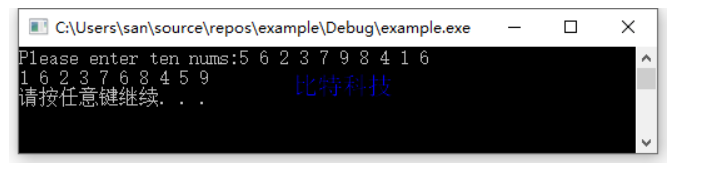4. 有n个整数,使前面各数顺序向后移m个位置,最后m个数变成最前面m个数,见图8.43。 写一函数实现以上功能,在主函数中输人n个整数和输出调整后的n个数。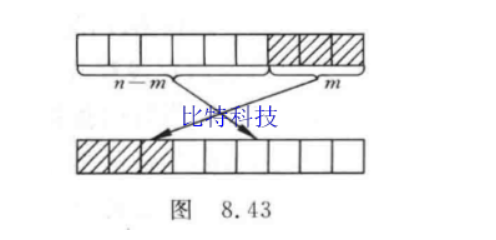解题思路： 找出倒数第m个数据，从这里开始保存倒数第m位置的数据，因为倒数第m位置的数据要放到数组最前方，将m之前的数据向后偏移一位，然后将数组第一个位置的数据替换为保存的m位的数据，逐个往后递增即可。
答案：
#include <stdio.h>
void move(int *arry, int n, int m)
{
int end_idx = n - m;//找到倒数第m个数据的位置，也就是要移动到数组首部的数据的起始位置
for (int i = 0; i < m; i++) {
int *p = arry + end_idx + i;//从倒数第m个数据的位置开始逐渐向后偏移直到数组尾部
int tmp = *p;//获取到这个位置的值，用于数组前边数据向后偏移1位之后，向数组第i位赋值
for (int j = end_idx + i; j > i; j--) {//从第i位开始逐个向后偏移一位
*p = *(p - 1);
p--;
}
*(arry + i) = tmp;//数组的前第i个数字逐个替换为后边被覆盖的值
}
}
int main()
{
int number, n, m, i;
printf("Please enter the number of numbers: ");
scanf_s("%d", &n);//先确定要输入多少个数字
printf("Please enter %d numbers: ", n);
for (i = 0; i < n; i++) {
scanf_s("%d", &number[i]);//输入指定个数的数字
}
printf("Number of positions to move: ");
scanf_s("%d", &m);//确定要向后移动多少个位置
move(number, n, m);
for (i = 0; i < n; i++) {
printf("%d ", number[i]);
}
printf("\n");
system("pause");
return 0;
}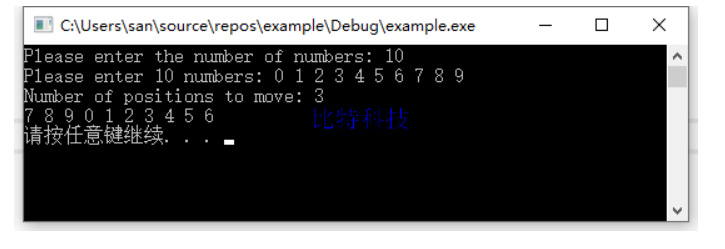5. 有n个人围成一圈，顺序排号。从第1个人开始报数(从1到3报数),凡报到3的人退出圈子,问最后留下的是原来第几号的那位。
解题思路： 从第一个人开始，逐个报数，谁报3就退出，并且重置报数，直到最后一个人后，又重新从第一个人继续报数，直到最终只剩一个人的时候退出即可。
答案：
#include <stdio.h>
int main()
{
int people, n;
printf("Please input how many people: ");
scanf_s("%d", &n);
for (int i = 0; i < n; i++) {
people[i] = i + 1; //对每个人顺序排号
}
int remain = n;
int num_off = 0;
int *p = NULL;
while (remain > 1) {
p = people;
while (p != people + n) { // 每次从第一个位置开始，直到最后一个位置,报数是一直递增的
if ((*p) != 0) {//若这个位置人还在
num_off++; //则报数
if (num_off == 3) {//否则当前的人即将要报的数字是3
*p = 0;  //则剔除这个人
num_off = 0; //并且重新开始计数，下边会++，所以是从1开始报数
remain--;//剩余人数-1
}
}
p++;
}
}
for (int i = 0; i < n; i++) {
if (people[i] != 0) {
printf("Serial number of the remaining person:%d\n", people[i]);
}
}

printf("\n");
system("pause");
return 0;
}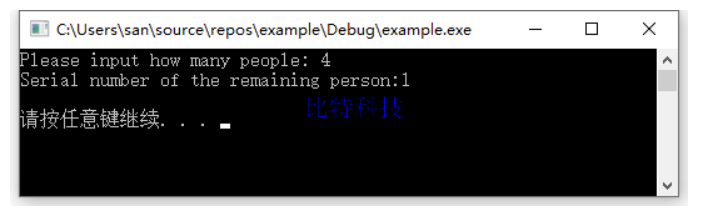6. 写一函数,求一个字符串的长度。在main函数中输入字符串,并输出其长度。
解题思路： 字符串以\0作为结尾，则从第一个字符开始向后移动遇到\0认为字符串结束。
答案：
#include <stdio.h>
int mystrlen(char *str)
{
int len = 0;
char *ptr = str;
while (*ptr != '\0') {
ptr++;
len++;
}
return len;
}
int main()
{
char buf;
printf("Please enter a string: ");
scanf_s("%s", buf, 1024);
printf("string len:%d\n", mystrlen(buf));
system("pause");
return 0;
}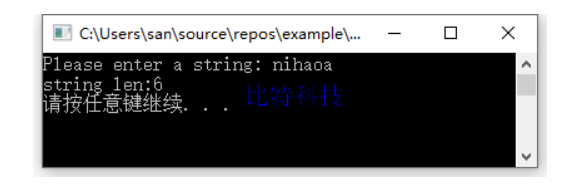7. 有一字符串,包含n个字符。写一函数,将此字符串中从第m个字符开始的全部字符复制成为另一个字符串。
解题思路： 当字符串指针移动到源字符串的第m位时，则开始向另一个缓冲区中写入剩下的数据
答案：
#include <stdio.h>
#include <string.h>

int main()
{
char buf1, buf2;
printf("Please enter a string: ");
scanf_s("%s", buf1, 1024);
int m;
printf("Please enter a location to start copying: ");
scanf_s("%d", &m);
if (m < 0 || m > strlen(buf1)) {//检测输入的位置是否合法
printf("Illegal location entered\n");
return -1;
}
char *ptr1 = buf1 + m; // 从第m个位置开始复制新数据
char *ptr2 = buf2;
while (*ptr1 != '\0') {
*ptr2++ = *ptr1++;
}
*ptr2 = '\0';//不要忘了字符串结尾标志
printf("%s\n", buf2);
system("pause");
return 0;
}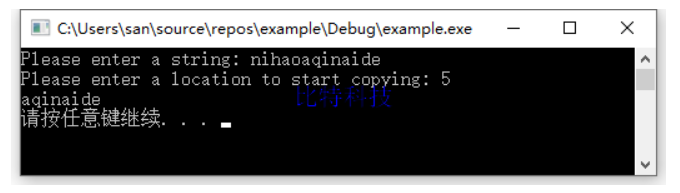8. 输入一行文字,找出其中大写字母、小写字母、空格、数字以及其他字符各有多少。
解题思路： 字符可以直接进行比较，但是要注意字符串中的数字是字符数字，必须以字符的形式比较，也就是加上单引号
答案：
#include <stdio.h>
#include <string.h>

int main()
{
char buf;
printf("Please enter a string: ");
gets_s(buf, 1024);
int upper_count = 0, lower_count = 0, digit_count = 0, space_count = 0, other_count = 0;
char *ptr = buf;
while (*ptr != '\0') {
if (*ptr >= 'A' && *ptr <= 'Z') { //大写字母
upper_count++;
}else if (*ptr >= 'a' && *ptr <= 'z'){//小写字母
lower_count++;
}else if (*ptr >= '0' && *ptr <= '9') {//数字字符
digit_count++;
}else if (*ptr== ' ') {//空格字符
space_count++;
}else { //其他字符
other_count++;
}
ptr++;
}
printf("upper:%d; lower:%d; digit:%d; space:%d; other:%d\n", \
upper_count, lower_count, digit_count, space_count, other_count);
system("pause");
return 0;
}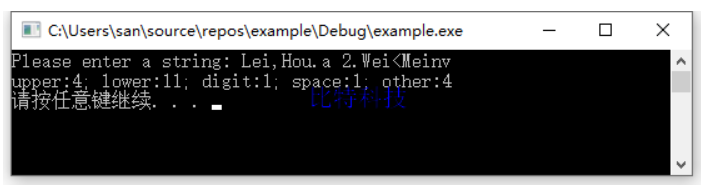9. 写一函数,将一个3x3的整型矩阵转置。
解题思路： 矩阵转置就是行变列，列变行，说白了就是 arry[i][j] 转换为 arry[j][i] ; 但是需要注意的是，
一. 因为行列个数可能并非相同，转换后行数或者列数变多了或者变少，因此不能直接转换。需要重新申请空间存储转换后的数据。
二. 二维数组是线性扁平化存储的，无法确定列数的情况下传参后，在函数中使用时需要头指针向后偏移 列数*第n行 才能访问到第n行的数据。例如在函数中访问 arry[i][j] ,需要通过arry + col_num*i + j 方式进行访问。
答案：
#include <stdio.h>
int **transform(int **arry, int row_count, int col_count)
{
//列变行，行变列，则行的个数是以前列的个数，列的个数是以前行的个数
int **p = NULL;
//矩阵中有多少行，取决于以前有多少列，然后申请地址空间
p = (int **)malloc(sizeof(int *) * col_count);
for (int i = 0; i < col_count; i++) {
//一行中有多少列，取决于以前有多少行，然后申请空间
p[i] = (int *)malloc(sizeof(int) * row_count);
}
for (int i = 0; i < col_count; i++) {
for (int j = 0; j < row_count; j++) {
//二维数组的存储是扁平化的， 访问第j行第i列的数据，应该是 arry + j*列数 + i
//j是新数组的列，但是是源数组的行
p[i][j] = (arry + col_count * j)[i];
}
}
return p;
}
int main()
{
int arry;
printf("Please enter a 3 x 4 matrix: \n");
for (int i = 0; i < 3; i++) {
for (int j = 0; j < 4; j++) {
scanf_s("%d", &arry[i][j]);
}
}
int **p = transform(arry, 3, 4);
printf("\n");
for (int i = 0; i < 4; i++) {
for (int j = 0; j < 3; j++) {
printf("%d ", p[i][j]);
}
free(p[i]); // 释放二级指针中每个一级指针申请的空间
printf("\n");
}
free(p);//释放总体二级指针的空间
system("pause");
return 0;
}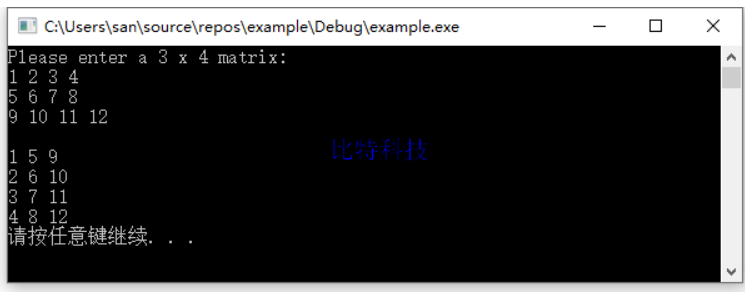10. 将一个5x5的矩阵中最大的元素放在中心,4个角分别放4个最小的元素(顺序为从左到右,从上到下依次从小到大存放),写一函数实现之。用main函数调用。
解题思路： 将二维数组当做一维数组进行处理比较方便，而二维数组本身就是扁平化存储，所以直接使用首地址即可。
先遍历找到最大值，将其与中间数字交换，而中间数字的下标就是数字总数除以2；
其次寻找四次最小值，每次寻找的时候将最小值的下标记录起来，前提是这个数字如果已经是选中的最小数字之一，则需要跳过，也就是只跟剩余的数字作比较。（第二次开始遍历找最小数字的时候，千万不能与第一个最小数进行比较，否则永远都只有一个最小数）。
答案：
#include <stdio.h>
#include <string.h>
void transform(int *arry, int col_row)
{
//找到最大值
int max = arry, max_idx;
for (int i = 0; i < col_row * col_row; i++) {
if (max < arry[i]) max = arry[i];//找出最大数
max_idx = i;
}
//行列相乘得到总数量，除以2后加1则为中心点（暂时不考虑偶数的情况）
int center_idx = (col_row * col_row) / 2;
int tmp = arry[cen ter_idx]; arry[center_idx] = arry[max_idx]; arry[max_idx] = tmp;

//找到四个最小值
int min_idx;
for (int i = 0; i < 4; i++) {//循环4次获取到最小值
int min_tmp = arry[col_row * col_row - 1];
for (int j = 0; j < col_row * col_row; j++) {//遍历所有数据，逐个比较获取最小值
int k = 0;
for (; k < i; k++) {//但是要注意如果某个下标的数据已经是获取过的最小值,则不能进行判断（因为这个肯定是最小的）
if (j == min_idx[k]) break;
}
if (k != i) { continue; }//k和i不同表示j这个坐标已经是找到的最小的几个数字之一，则找下一个判断
if (min_tmp > arry[j]) { // 相当于在剩下的数中找到最小的那个数字
min_tmp = arry[j];
min_idx[i] = j; //并且记录这个数字的位置
}
}
}
int change_idx;//先计算四个角的下标，便于后边进行交换
change_idx = 0;//第一个要置换的数据的下标，也就是左上角
change_idx = col_row - 1;//第二个要置换的数据的下标，也就是右上角
change_idx = col_row * (col_row - 1);//第一个要置换的数据的下标，也就是左下角
change_idx = (col_row * col_row) - 1;//第一个要置换的数据的下标，也就是右下角
for (int i = 0; i < 4; i++) {
int tmp = arry[change_idx[i]]; arry[change_idx[i]] = arry[min_idx[i]]; arry[min_idx[i]] = tmp;
}
return ;
}
int main()
{
int arry;
printf("Please enter a 5x5 matrix: \n");
for (int i = 0; i < 5; i++) {
for (int j = 0; j < 5; j++) {
scanf_s("%d", &arry[i][j]);
}
}
transform(*arry, 5);//将二维数组当做一维数组传入处理，并且传入行列数
printf("\n");
for (int i = 0; i < 5; i++) {
for (int j = 0; j < 5; j++) {
printf("%d ", arry[i][j]);
}
printf("\n");
}
system("pause");
return 0;
}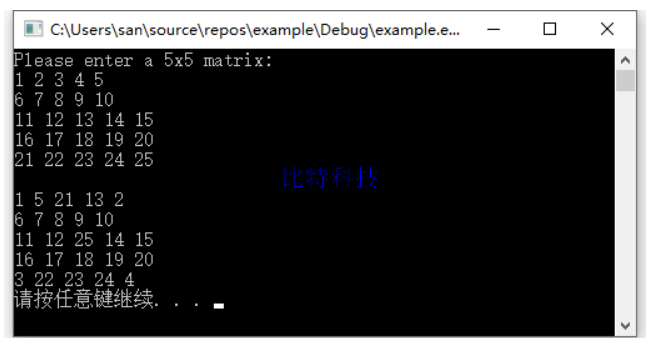11. 在主函数中输入10个等长的字符串。用另一函数对它们排序。然后在主函数输出这10个已排好序的字符串。
解题思路： 排序方式与数字比较没什么不同，先遍历比较找出最大的字符串，与第一个字符串进行交换，然后剩下的进行比较找出最大的字符串与第二个交换…
需要主机的就是字符串的比较采用strcmp接口，返回值大于0表示第一个字符串大于第二个字符串
答案：
#include<stdio.h>
#include<string.h>
void sort(char s)
{
int i, j;
for (i = 0; i < 10; i++){
for (j = i; j < 10; j++){
if (strcmp(s[i], s[j])> 0){
char tmp;
strcpy_s(tmp, 32, s[i]);
strcpy_s(s[i], 32, s[j]);
strcpy_s(s[j], 32, tmp);
}
}
}
}
int main()
{
char str;
printf("Please enter ten strings:\n");
for (int i = 0; i < 10; i++){
scanf_s("%s", str[i], 32);
}
sort(str);
printf("\n");
for (int i = 0; i < 10; i++){
printf("%s\n", str[i]);
}
system("pause");
return 0;
}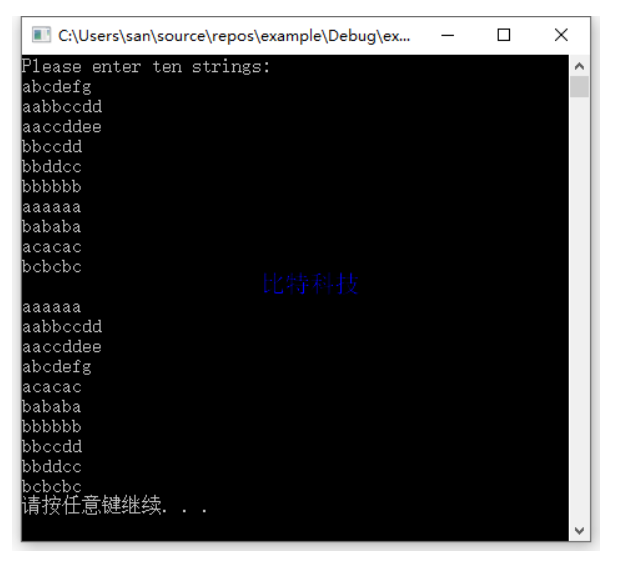12. 用指针数组处理上一题目,字符串不等长。
解题思路： 与数字的比较没什么区别，只不过需要采用strcmp进行字符串的大小比较，使用指针实现需要在最早接收数据的时候就采用字符串指针数组，这样的好处在于指针的指向可以直接通过赋值进行改变，而指针数组中的字符串的顺序只需要交换不同字符串的地址就可以实现
答案：
#include<stdio.h>
#include<string.h>
void sort(char *s)
{
int i, j;
for (i = 0; i < 10; i++){
for (j = i; j < 10; j++){
if (strcmp(s[i], s[j])> 0){
char *tmp = s[i]; //指针的好处在于直接通过赋值可以改变指向
s[i] = s[j];  //只要交换了字符串的地址就实现了字符串的交换
s[j] = tmp;//因此通过指针指向的交换就能够实现数组中字符串的排序
}
}
}
}
int main()
{
char *str;
printf("Please enter ten strings:\n");
for (int i = 0; i < 10; i++) {
str[i] = malloc(32);//为每一个指针分配空间
scanf_s("%s", str[i], 32);
}
sort(str);
printf("\n");
for (int i = 0; i < 10; i++){
printf("%s\n", str[i]);
free(str[i]);
}
system("pause");
return 0;
}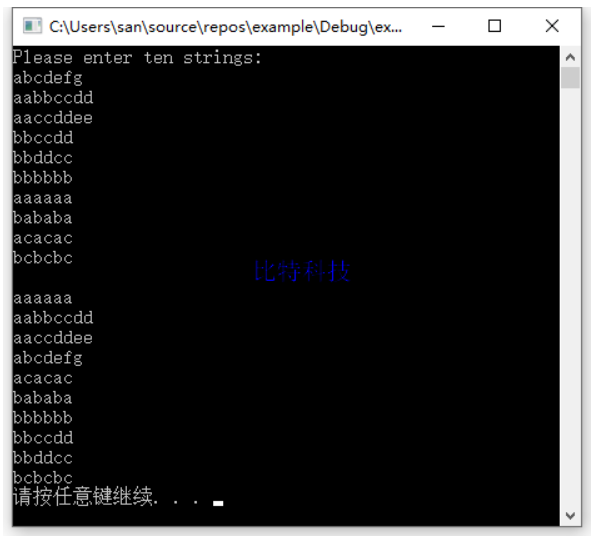13. 写一个用矩形法求定积分的通用函数,分别求$\int_0^1 sinxdx, \quad \int_0^1 cosxdx, \quad \int_0^1 e^xdx,$ ,说明: sin,cos,exp 函数已在系统的数学函数库中，程序开头要用#include <math. h>。
解题思路：
矩形法，学过高等数学就知道化曲为直的思想。将定积分化为多个函数连续的和。基本思想是将区间[a，b]化成n等分，当n越大的时候结果越准确。图形化成一小块一小块的矩形。底边长都为(b-a)/n.高为每个等分点的函数值。然后将每个矩形的面积相加即为所求。
因为被分成n等分，就可以认为每一等分是一个矩形，那么每一矩形的面积为： 每一个矩形面积为：
Sn=f(x)(b-a)/n ；总面积为：S=S1+S2+…+Sn；具体计算过程根据公式套即可
这里主要在于函数指针的应用，将函数作为参数传递给另一个函数，在另一个函数中进行调用的方式向外提供统一接口，而接口内的处理方式随着传入的函数而不同。
答案：
#include<stdio.h>
#include<math.h>

double integral(double(*handler)(double), double a, double b, int n)
{
double i,s = 0;
double h = (b - a) / n;
for (i = a; i <= b; i += h){
s += handler(i) * h;
}
return s;
}
int main()
{
double a, b;
int n = 200000; // 区间划分个数
int func_idx; // 计算函数选择id
printf("Please enter the lower and upper limit of integration:");
scanf_s("%lf %lf", &a, &b);
printf("Please enter specific calculation function(1-sin/2-cos/3-exp): ");
scanf_s("%d", &func_idx);
switch (func_idx) {
case 1:printf("The integral of sin(x) is:%lf\n", integral(sin, a, b, n)); break;
case 2:printf("The integral of cos(x) is:%lf\n", integral(cos, a, b, n)); break;
case 3:printf("The integral of exp(x) is:%lf\n", integral(exp, a, b, n)); break;
default:
printf("function id error\n");
return -1;
}
system("pause");
return 0;
}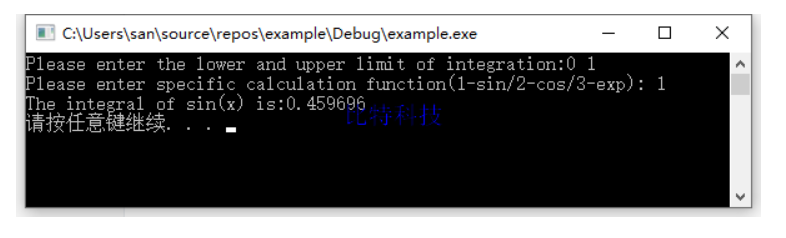14. 将n个数按输入时顺序的逆序排列,用函数实现。
解题思路： 定义两个指针，一个指向数组头部，一个指向数组尾部，头部每次+1，尾部每次-1；则在头部小于尾部的情况下进行数据交换即可。
答案：
#include<stdio.h>
#include<math.h>

void reorder(int *arry, int n)
{
int *start = arry;
int *end = arry + n - 1;
for (; start < end; start++, end--) {
int tmp = *start;
*start = *end;
*end = tmp;
}
return;
}

int main()
{
int arry;
printf("Please enter ten numbers:");
for (int i = 0; i < 10; i++) {
scanf_s("%d", &arry[i]);
}
reorder(arry, 10);
printf("\n");
for (int i = 0; i < 10; i++) {
printf("%d ", arry[i]);
}
printf("\n");
system("pause");
return 0;
}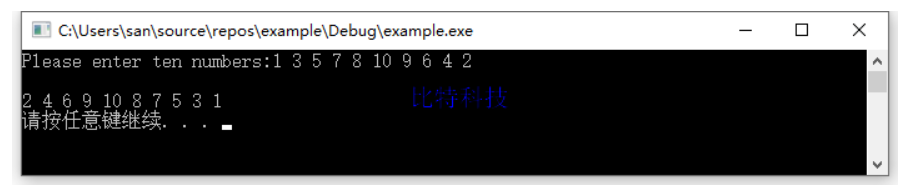15. 有一个班4个学生,5门课程。
①求第1门课程的平均分;
②找出有两门以上课程不及格的学生,输出他们的学号和全部课程成绩及平均成绩;
③找出平均成绩在90分以上或全部课程成绩在85分以上的学生。
分别编3个函数实现以上3个要求。
解题思路： 4个学生，5门课程就是一个4x5的二维数组，

求第一门课的平均分，就是将第0列数据相加除以学生人数
遍历每个学生的成绩，判断不及格次数，若大于2则输出信息即可
统计每个学生总成绩，以及对每门课程成绩进行判断即可

答案：
#include<stdio.h>
#include<math.h>

float avg(int arry[], int n)
{
float sum = 0;
for (int i = 0; i < n; i++) {
sum += arry[i];
}
printf("Average of course 1:%f\n", (sum / n));
return (sum / n);
}
void fail(int arry[], int n)
{
printf("Information on students who fail in more than two courses: ");
for (int i = 0; i < n; i++) {
int sum = 0, fail_count = 0;
for (int j = 0; j < 5; j++) {
if (arry[i][j] < 60) {
fail_count++;
}
}
if (fail_count <= 2) {
continue;
}
printf("seq:%d ", i + 1);
printf("score: ");
for (int j = 0; j < 5; j++) {
sum += arry[i][j];
printf("%d ", arry[i][j]);
}
printf("avg:%d ", sum / 5);
printf("\n");
}
return;
}
void excellent(int arry[], int n)
{
int i, j;
for (i = 0; i < n; i++) {
int sum = 0, count = 0;
for (j = 0; j < 5; j++) {
sum += arry[i][j]; //计算总分用于计算平均分
if (arry[i][j] > 85) {
count++; //若每门课都大于85则count总会与j同步++
}
}
if ((sum / 5) > 90 || count == j) {
printf("Excellent students: %d\n", i + 1);
}
}
return;
}

int main()
{
int arry;
printf("Please enter a 4x5 matrix:\n");
for (int i = 0; i < 4; i++) {
for (int j = 0; j < 5; j++) {
scanf_s("%d", &arry[i][j]);
}
}
avg(arry, 4);
fail(arry, 4);
excellent(arry, 4);
printf("\n");
system("pause");
return 0;
}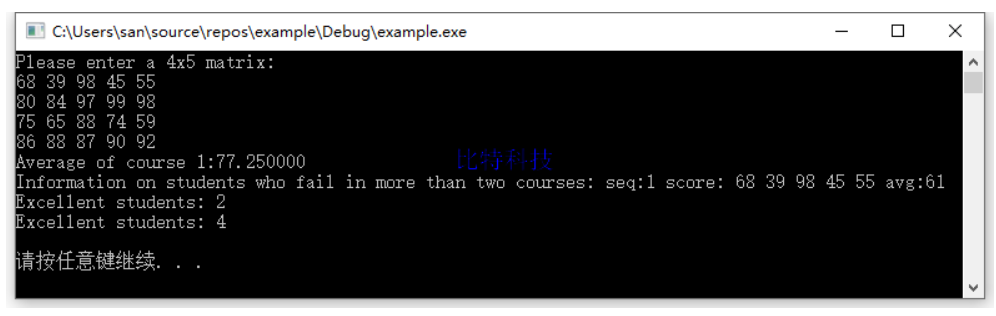16. 输入一个字符串,内有数字和非数字字符,例如：A123x456 17960? ,302tab5876，将其中连续的数字作为一个整数,依次存放到一数组a中。例如,123放在a,456放在a1…统计共有多少个整数,并输出这些数。
解题思路： 遇到数字字符，则开始统计连续数字字符的个数，统计完毕后，则从右往左每次乘以10（除了个位数）作为数字的单位，最终相加得到数字；然后越过这个数字，从后边继续查找。
答案：
#include<stdio.h>
#include<string.h>

void count_digit(char *str)
{
int digit, count = 0;
char *ptr = str;
int i = 0, str_len = strlen(str);
while (i < str_len) {
if (*(ptr+i) >= '0' && *(ptr+i) <= '9') {
int len = 1;//用于统计连续数字的个数
while (*(ptr + i + len) >= '0' && *(ptr + i+ len) <= '9' && (i+len) < str_len) {//找出从当前位置连续数字的个数
len++;
}
int sum = *(ptr + i + len - 1) - '0';//先获取个位数的数据
int unit = 1;//每一位的单位，从十位开始每次乘以10作为单位
for (int j = len - 2; j >= 0; j--) {//从右往左逐个处理
unit *= 10;
sum += (*(ptr + i + j) - '0') * unit;
}
digit[count++] = sum;
i += len; // i需要加上len的长度，越过这个数字，防止一个连续数字中的字符重复处理
continue;
}
i++;
}
for (int i = 0; i < count; i++) {
printf("%d ", digit[i]);
}
return;
}
int main()
{
char buf = { 0 };
printf("Please enter a string with numbers:\n");
gets_s(buf, 1024);
count_digit(buf);
printf("\n");
system("pause");
return 0;
}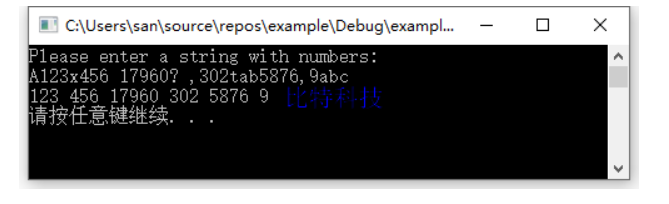17. 写一函数，实现两个字符串的比较。即自己写一个strcmp函数,函数原型为int strcmp(char * p1 ,char * p2); 设p1指向字符串s1, p2指向字符串s2。要求当s1=s2时,返回值为0;若s1≠s2,返回它们二者第1个不同字符的ASCII码差值(如"BOY"与"BAD" ,第2个字母不同,0与A之差为79- 65=14)。如果s1>s2,则输出正值;如果s1<s2,则输出负值。
解题思路： 使用两个指针指向两个字符串首部，逐个往后进行比较，不相等的时候则将数据进行相减，作为返回值。
答案：
#include<stdio.h>
#include<string.h>

int mystrcmp(char *str1, char *str2)
{
char *ptr1 = str1;
char *ptr2 = str2;
int res;
while (*ptr1 != '\0' && *ptr2 != '\0') {
if (*ptr1 != *ptr2) {
res = *ptr1 - *ptr2;
break;
}
ptr1++;
ptr2++;
}
if (*ptr1 == '\0' || *ptr2 == '\0') {//注意一个字符串到达结尾或者两个都到达结尾的情况
res = *ptr1 - *ptr2;
}
return res;
}
int main()
{
char buf1 = { 0 };
char buf2 = { 0 };
while (1) {
printf("Please enter two strings:\n");
gets_s(buf1, 1024);
gets_s(buf2, 1024);
printf("mystrcmp:%d", mystrcmp(buf1, buf2));
printf("\n");
}
system("pause");
return 0;
}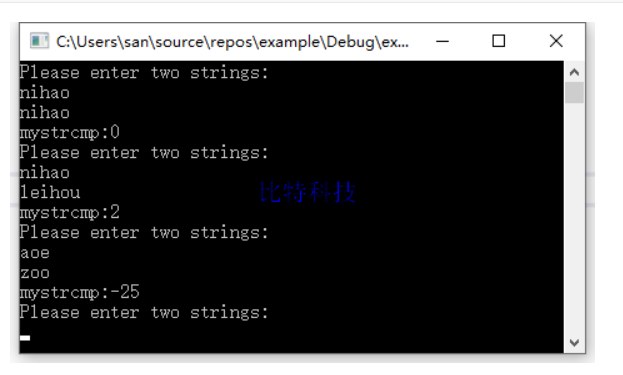18. 编一程序,输入月份号,输出该月的英文月名。例如，输人3,则输出"March" ,要求用指针数组处理。
解题思路： 首先定义字符串指针数字，数组中每一个元素都存放一个字符串指针，每个指针指向不同字符串的位置。则输入月份数字后，根据下标获取对应月份字符串的地址即可
答案：
#include<stdio.h>
int main()
{
int month;
char* Month = { "January","February","March","April","May","June",
"July","August","September","October","November","December" };
while (1) {
printf("Please enter the month: ");
scanf_s("%d", &month);
if (month < 1 && month>12) {
printf("Input error, Month should be greater than 0 and less than 12\n");
}
printf("%s\n", Month[month - 1]);
}
return 0;
}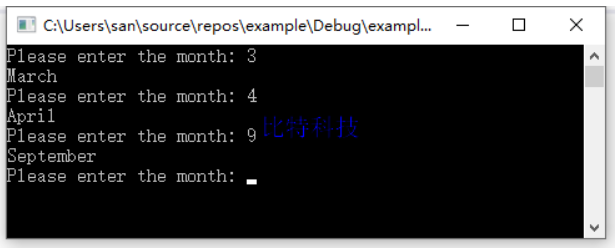19.(1) 编写一个函数new,对n个字符开辟连续的存储空间,此函数应返回一个指针(地址),指向字符串开始的空间。new(n)表示分配n个字节的内存空间。(2)写一函数free,将前面用new函数占用的空间释放。free§表示将p(地址)指向的单元以后的内存段释放。
解题思路： 封装malloc函数申请空间，封装free函数释放空间；
答案：
#include <stdio.h>
#include <stdlib.h>

void *mynew(int n)
{
return malloc(n);
}
void myfree(char *p)
{
return free(p);
}
int main()
{
int num;
char *str = NULL;
printf("Please enter number: ");
scanf_s("%d", &num);
printf("before new p--%p:%s\n", str, str);//申请空间之前，查看指针的地址和指向空间数据
str = (char*)mynew(num);
printf("after new p--%p:%s\n", str, str);//申请空间之后，查看指针的地址和指向空间数据
printf("Please enter a string:");
scanf_s("%s", str, num);
printf("before free p--%p:%s\n", str, str);//释放空间之前，查看指针的地址和指向空间数据
myfree(str);
printf("after free p--%p:%s\n", str, str);//释放空间之后，查看指针的地址和指向空间数据
system("pause");
return 0;
}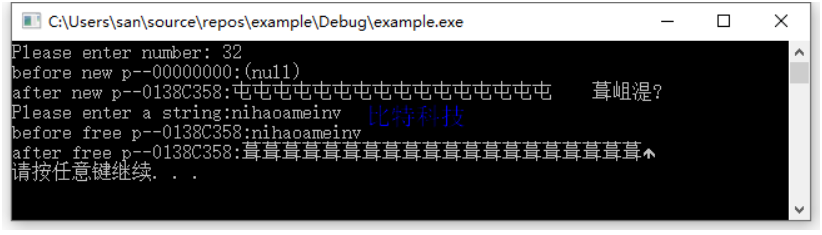20. 用指向指针的指针的方法对5个字符串排序并输出。
**解题思路：**指向指针的指针其实就是二级指针，使用二级指针的保存一级指针的地址，让这个一级指针指向具体的数据空间； 定义一个字符串指针数组包含5个元素，每个元素可以保存一个字符串的首地址，而这个地址指向的是具体的字符串数据空间，通过指针访问实现对指向空间内的字符串进行比较排序交换即可。
答案：
#include<stdio.h>
#include<string.h>
void sort(char *s)
{
int i, j;
for (i = 0; i < 10; i++){
for (j = i; j < 10; j++){
if (strcmp(s[i], s[j])> 0){//s[i]保存的就是一个字符串的首地址
char *tmp = s[i]; //指针的好处在于直接通过赋值可以改变指向
s[i] = s[j];  //只要交换了字符串的地址就实现了字符串的交换
s[j] = tmp;//因此通过指针指向的交换就能够实现数组中字符串的排序
}
}
}
}
int main()
{
char *str;//定义字符串指针数组，可以分别保存指向5个字符串所在空间首地址
printf("Please enter five strings:\n");
for (int i = 0; i < 5; i++) {
str[i] = malloc(32);//为每一个指针分配空间
scanf_s("%s", str[i], 32);
}
sort(str);
printf("\n");
for (int i = 0; i < 5; i++){
printf("%s\n", str[i]);
free(str[i]);
}

system("pause");
return 0;
}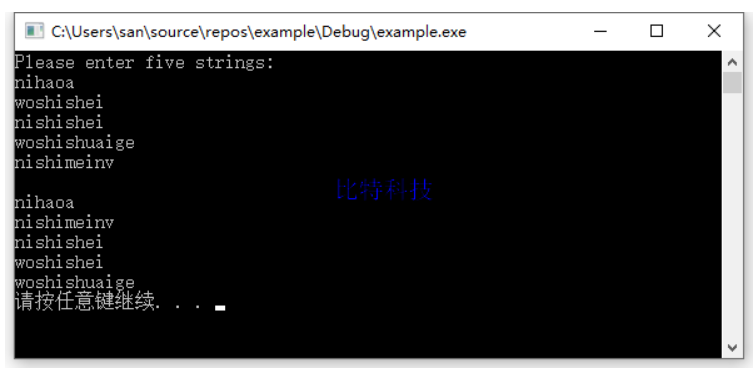21. 用指向指针的指针的方法对n个整数排序并输出。要求将排序单独写成一个函数。n个整数在主函数中输入,最后在主函数中输出。
解题思路： 指向指针的指针其实就是二级指针，使用二级指针的保存一级指针的地址，让这个一级指针指向具体的数据空间。排序就是按照每个元素指针指向空间中的数据进行比对排序即可。
答案：
#include<stdio.h>
#include<string.h>
void sort(int *s[], int len)
{
int i, j;
for (i = 0; i < len; i++) {
for (j = i; j < len; j++) {
//s[i]就是s指针数组中第i个元素保存的一级指针，再次解引用就是最终的数据空间
if (*(s[i]) > *(s[j])) {
int tmp = *(s[i]); //指针的好处在于直接通过赋值可以改变指向
*(s[i]) = *(s[j]);  //只要交换了字符串的地址就实现了字符串的交换
*(s[j]) = tmp;//因此通过指针指向的交换就能够实现数组中字符串的排序
}
}
}
}
int main()
{
int *integers;
int count = 0;
printf("Please enter the number of count: ");
scanf_s("%d", &count);
integers = (int*)malloc(count * sizeof(int));//为指针申请count个int空间用于存放输入的数据
int **p = (int **)malloc(count * sizeof(int*));//为指针申请count个int*空间用于存放int空间的首地址
printf("Please enter %d integers: \n", count);
for (int i = 0; i < count; i++) {
p[i] = integers + i; //将数组中每个int元素空间的首地址赋值给指针数组中的各个元素
scanf_s("%d", p[i]);//p[i]就保存时第i个数据的地址，因此这里不用取地址
}
sort(p, count);
for (int i = 0; i < count; i++) {
printf("%d ", integers[i]);
}
printf("\n");
free(integers);
free(p);
system("pause");
return 0;
}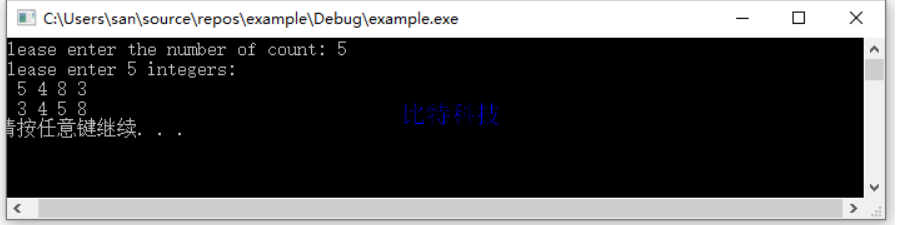这个B站上的视频c语言从入门到进阶，老师讲的相当好

C语言程序设计第五版谭浩强更多习题答案
C语言程序设计第五版谭浩强 第七章答案
C语言程序设计第五版谭浩强 第九章答案


展开全文• C语言程序设计谭浩强第五版课后答案第三章 1、假如我国国民生产总值的年增长率为7%， 计算10年后我国国民生产总值与现在相比增长多少百分比。计算公式为p=(1+r)np = (1+r)^np=(1+r)n ,其中r为年增长率，n为年数，p为...
C语言程序设计谭浩强第五版课后答案第三章1、假如我国国民生产总值的年增长率为7%， 计算10年后我国国民生产总值与现在相比增长多少百分比。计算公式为$p = (1+r)^n$ ,其中r为年增长率，n为年数，p为与现在相比的倍数。
题目解析:

此题的关键主要是利用数学库math中pow函数进行计算，若不熟悉可以查阅帮助文档，查看pow函数的用法。

代码示例:
#include<stdio.h>
#include <math.h>
int main()
{
float p, r, n;
r = 0.07;
n = 10;
p = pow(1 + r, n);
printf("p=%f\n", p);
return 0;
}

运行结果: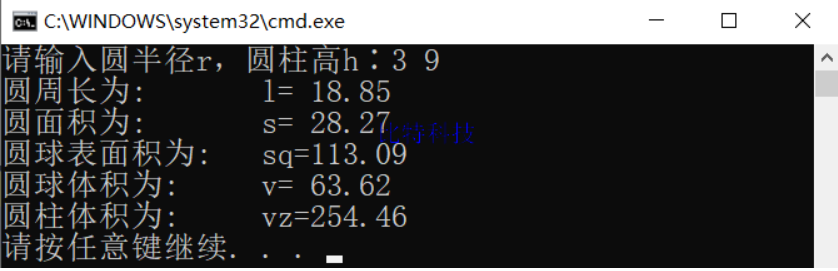2、存款利息的计算。有1000元，想存5年，可按以下5种办法存：
（1）一次存5年期
（2）先存2年期，到期后将本息再存3年期
（3）先存3年期，到期后将本息再存2年期
（4）存1年期，到期后将本息再存1年期，连续存5次
（5）存活期存款，活期利息每一季度结算一次
2017年银行存款利息如下：

1年期定期存款利息为1.5%;

2年期定期存款利息为2.1%;

3年期定期存款利息为2.75%;

5年期定期存款利息为3%;

活期存款利息为0.35%(活期存款每一-季度结算一-次利息)

如果r为年利率，n为存款年数，则计算本息的公式如下：

1年期本息和: P= 1000* (1+r);

n年期本息和: P= 1000* (1+n* r);

存n次1年期的本息和: $P=1000* (1+r)^n$;

活期存款本息和: P= 1000 *(1+$\frac{r}{4}$)$^{4n}$;

说明: 1000*(1+$\frac{r}{4}$)是一个季度的本息和。
题目解析:
理解题意很关键，其次就是利用数学库math中pow函数进行幂次方计算
代码示例:
#include<stdio.h>
#include <math.h>

int main()
{
float r5, r3, r2, r1, r0, p, p1, p2, p3, p4, p5;
p = 1000;
r5 = 0.0585;
r3 = 0.054;
r2 = 0.0468;
r1 = 0.0414;
r0 = 0.0072;

p1 = p*((1 + r5) * 5);                // 一次存5年期
p2 = p*(1 + 2 * r2)*(1 + 3 * r3);     // 先存2年期，到期后将本息再存3年期
p3 = p*(1 + 3 * r3)*(1 + 2 * r2);     // 先存3年期，到期后将本息再存2年期
p4 = p*pow(1 + r1, 5);                // 存1年期，到期后将本息存再存1年期，连续存5次
p5 = p*pow(1 + r0 / 4, 4 * 5);        // 存活期存款。活期利息每一季度结算一次
printf("p1=%f\n", p1);       // 输出按第1方案得到的本息和
printf("p2=%f\n", p2);       // 输出按第2方案得到的本息和
printf("p3=%f\n", p3);       // 输出按第3方案得到的本息和
printf("p4=%f\n", p4);       // 输出按第4方案得到的本息和
printf("p5=%f\n", p5);       // 输出按第5方案得到的本息和
return 0;
}

运行结果: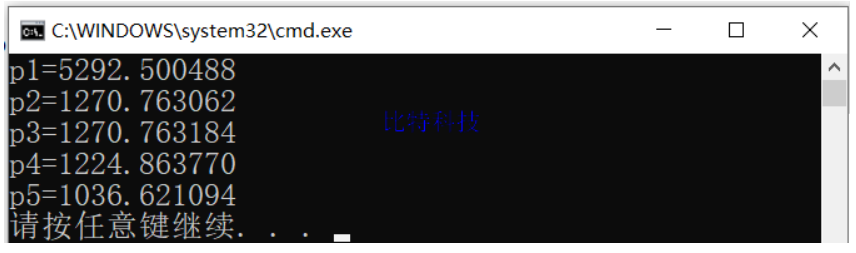3、购房从银行贷了一笔款d,准备每月还款额为p,月利率为r,计算多少月能还清。设d为300 000元,p为6000元,r为1%。对求得的月份取小数点后一位,对第2位按四舍五人处理。
提示:计算还清月数m的公式如下: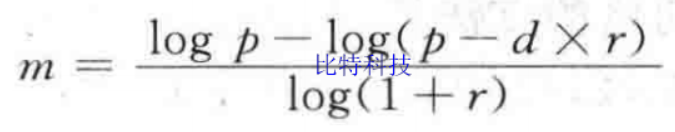可以将公式改写为: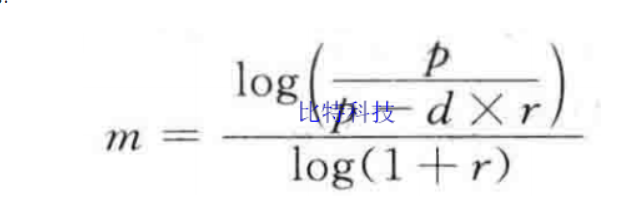C的库函数中有求对数的函数log10,是求以10为底的对数,log( p )表示log p。
题目解析:
该题的关键主要是利用数学函数库中的log函数进行求解，然后月份要求按照小数点后一位进行四舍五入进行处理，只需要在输出时进行格式控制即可。
代码示例:
#include<stdio.h>
#include <math.h>

int main()
{
float d = 300000, p = 6000, r = 0.01, m;
m = log10(p / (p - d*r)) / log10(1 + r);
printf("m = %3.1f\n", m);
return 0;
}

运行结果: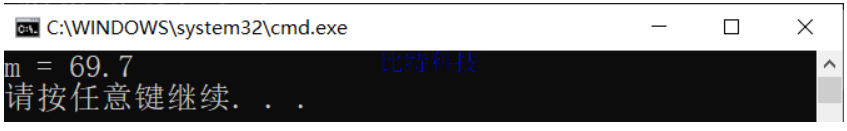4、分析下面的程序:
#include<stdio.h>
int main()
{
char c1, c2;
c1 = 97;
c2 = 98;
printf("c1=%c, c2=%c\n", c1, c2);
printf("c1=%d, c2=%d\n", c1, c2);
return 0;
}

(1)运行时会输出什么信息?为什么?
​     答：会输出 ：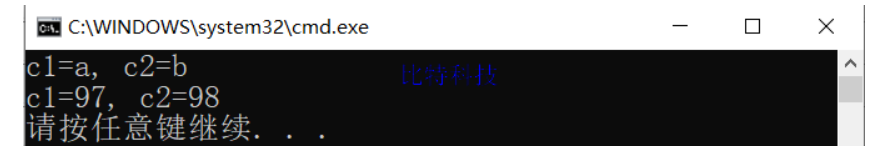​                          c1=97, c2=98

因为第一行十一字符形式输出，所以会吧97和98当作相依字符的ascii值，输出的时候按照字符显示，

第二行由于是以整形输出，所以输出对应整数值

(2)如果将程序第4,5行改为

c1 = 197;

c2 = 198;

运行时会输出什么信息?为什么?
​    答: 会输出：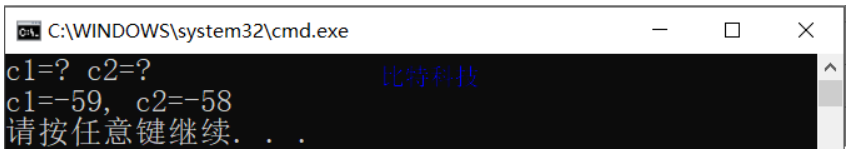因为c1和c2为char类型，其保存的数据范围为-128~127，当c1=197,c2=198时，已经超出了char类型所能够保存的数据范围，此时会进行相应的转换为c1=-59,c2=-58，只是第一行以字符输出时，c1=-59,c2=-58为不可显示字符，所以输出了？,而第二行以整数输出时就进行了相应的整数显示。

(3)如果将程序第3行改为
int c1, c2;
运行时会输出什么信息?为什么?
​    答: 会输出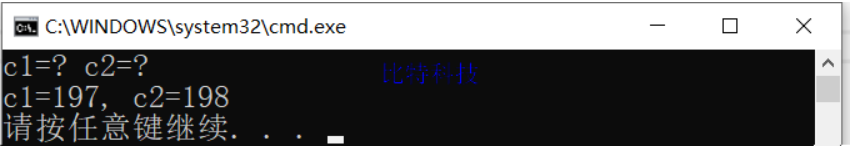因为c1和c2为int时，第二行以整形输出自然能够保存的下，但第一行以字符输出时，虽然数据int能够储存，但是在输出时需要准换为字符，转换后数据就超出了范围，此时c1=-59,c2=-58，两者都为不可显示字符，所以就显示了？。

5、用下面的scanf 函数输人数据,使a=3,b=7,x=8.5,y=71. 82,cl=‘A’,c2=‘a’。在键盘上应如何输入?
#include<stdio.h>
int main()
{
int a, b;
float x, y;
char c1, c2;
scanf("a=%db=%d", &a, &b);
scanf("%f%e",&x, &y);
scanf("%c%c",&c1, &c2);
return 0;
}

题目解析:

此题主要考察格式化输入，整形输入a和b时，必须按照a=3b=7的格式输入，浮点数输入时，%e代表科学记数法输入，字符输入c1和c2时，中间不能有任何空格

运行结果: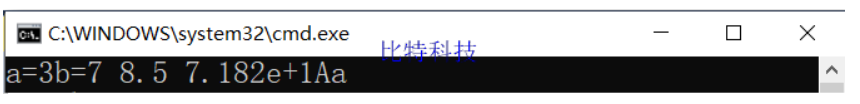6、请编程序将“China"译成密码，密码规律是:用原来的字母后面第4个字母代替原来的字母。例如,字母“A”后面第4个字母是“E”,用“E”代替“A”。因此,“China"应译为“Glmre”。请编一程序,用赋初值的方法使cl,c2,c3,c4,c5这5个变量的值分别为’C’,‘h’,‘i’,‘n’,‘a’ ,经过运算,使c1,c2,c3,c4,c5 分别变为’G’,‘l’,‘m’,‘r’,‘e’。分别用putchar函数和printf函数输出这5个字符。
题目解析:

密码转换的关键在于相应字符的ascii加4进行赋值修改原来的字符即可，修改完成后即为相应的密码，在使用putchar和printf进行相应输出即可。

代码示例:
#include<stdio.h>

int main()
{
char c1 = 'C', c2 = 'h', c3 = 'i', c4 = 'n', c5 = 'a';
c1 = c1 + 4;
c2 = c2 + 4;
c3 = c3 + 4;
c4 = c4 + 4;
c5 = c5 + 4;

//使用putchar输出
printf("使用putchar输出: ");
putchar(c1);
putchar(c2);
putchar(c3);
putchar(c4);
putchar(c5);
printf("\n");

//使用printf输出
printf("使用putchar输出: %c%c%c%c%c\n", c1, c2, c3, c4, c5);
return 0;
}

运行结果: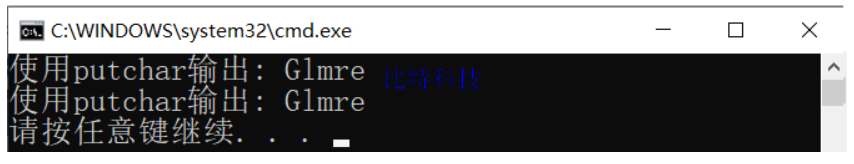7、设圆半径r=1.5,圆柱高h=3,求圆周长、圆面积、圆球表面积、圆球体积、圆柱体积。用scanf输人数据，输出计算结果，输出时要求有文字说明,取小数点后2位数字。请编程序。
题目解析:
此题主要理解圆柱的各种求法，其次要求取小数点后两位，只需在打印时进行格式控制即可。
代码示例:
#include<stdio.h>

int main()
{
float h, r, l, s, sq, vq, vz;
float pi = 3.141526;
printf("请输入圆半径r，圆柱高h∶");
scanf("%f %f", &r, &h);               //要求输入圆半径r和圆柱高h
l = 2 * pi*r;                          //计算圆周长l
s = r*r*pi;                          //计算圆面积s
sq = 4 * pi*r*r;                       //计算圆球表面积sq
vq = 3.0 / 4.0*pi*r*r*r;               //计算圆球体积vq
vz = pi*r*r*h;                       //计算圆柱体积vz
printf("圆周长为:       l=%6.2f\n", l);
printf("圆面积为:       s=%6.2f\n", s);
printf("圆球表面积为:   sq=%6.2f\n", sq);
printf("圆球体积为:     v=%6.2f\n", vq);
printf("圆柱体积为:     vz=%6.2f\n", vz);
return 0;
}


运行结果: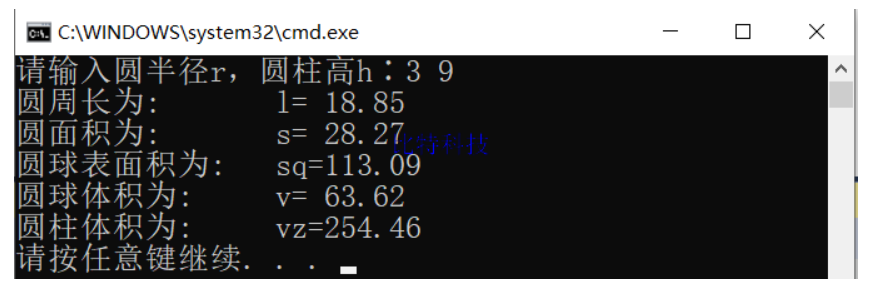8.编程序,用getchar函数读人两个字符给c1和c2，然后分别用putchar函数和printf函数输出这两个字符。思考以下问题:
(1) 变量cl和c2应定义为字符型、整型还是二者皆可?
(2) 要求输出cl和c2值的ASCII码,应如何处理?用putchar函数还是printf函数?
(3) 整型变量与字符变量是否在任何情况下都可以互相代替?如:
char c1,c2;
与
int cl,c2;
是否无条件地等价?
题目解析:
(1) 变量cl和c2应定义为字符型、整型还是二者皆可?

答：c1和c2 应定义为整形，因为定义为字符有可能返回的整数会超出字符的存储范围

(2) 要求输出cl和c2值的ASCII码,应如何处理?用putchar函数还是printf函数?

答：需要使用printf函数，打印是以整形形式打印

(3) 整型变量与字符变量是否在任何情况下都可以互相代替

答：不是，因为两者的存储范围不同，如果整数超出了字符的存储范围，则不能相互替换

代码示例:
int main()
{
int c1, c2;
printf("请输入两个字符c1,c2:");
c1 = getchar();
c2 = getchar();
printf("用putchar语句输出结果为:");
putchar(c1);
putchar(c2);
printf("\n");
printf("用printf语句输出结果为:");
printf("%c %c\n", c1, c2);
return 0;
}

运行结果: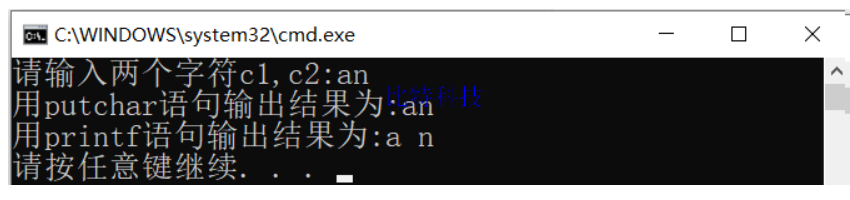更多
C语言程序设计谭浩强第五版课后答案 第二章习题答案
C语言程序设计谭浩强第五版课后答案 第四章习题答案


展开全文• c程序设计第五版谭浩强课后习题答案 第三章 最简单的C程序设计 ----顺序程序设计 1、假如我国国民生产总值的年增长率为7%， 计算10年后我国国民生产总值与现在相比增长多少百分比。计算公式为p=(1+r)np = (1+r)^np=...
c程序设计第五版谭浩强课后习题答案
第三章  最简单的C程序设计 ----顺序程序设计
1、假如我国国民生产总值的年增长率为7%， 计算10年后我国国民生产总值与现在相比增长多少百分比。计算公式为$p = (1+r)^n$ ,其中r为年增长率，n为年数，p为与现在相比的倍数。
题目解析:
此题的关键主要是利用数学库math中pow函数进行计算，若不熟悉可以查阅帮助文档，查看pow函数的用法。
代码示例:
#include<stdio.h>
#include <math.h>
int main()
{C
float p, r, n;
r = 0.07;
n = 10;
p = pow(1 + r, n);
printf("p=%f\n", p);
return 0;
}

运行结果: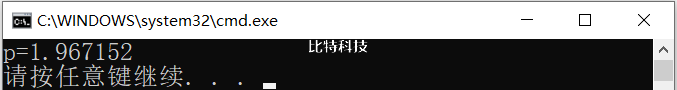2、存款利息的计算。有1000元，想存5年，可按以下5种办法存：
（1）一次存5年期
（2）先存2年期，到期后将本息再存3年期
（3）先存3年期，到期后将本息再存2年期
（4）存1年期，到期后将本息再存1年期，连续存5次
（5）存活期存款，活期利息每一季度结算一次
2017年银行存款利息如下：
1年期定期存款利息为1.5%;
2年期定期存款利息为2.1%;
3年期定期存款利息为2.75%;
5年期定期存款利息为3%;
活期存款利息为0.35%(活期存款每一-季度结算一-次利息)
如果r为年利率，n为存款年数，则计算本息的公式如下：
1年期本息和: P= 1000* (1+r);
n年期本息和: P= 1000* (1+n* r);
存n次1年期的本息和: $P=1000* (1+r)^n$;
活期存款本息和: P= 1000 *(1+$\frac{r}{4}$)$^{4n}$;
说明: 1000*(1+$\frac{r}{4}$)是一个季度的本息和。
题目解析:
理解题意很关键，其次就是利用数学库math中pow函数进行幂次方计算
代码示例:
#include<stdio.h>
#include <math.h>

int main()
{
float r5, r3, r2, r1, r0, p, p1, p2, p3, p4, p5;
p = 1000;
r5 = 0.0585;
r3 = 0.054;
r2 = 0.0468;
r1 = 0.0414;
r0 = 0.0072;

p1 = p*((1 + r5) * 5);                // 一次存5年期
p2 = p*(1 + 2 * r2)*(1 + 3 * r3);     // 先存2年期，到期后将本息再存3年期
p3 = p*(1 + 3 * r3)*(1 + 2 * r2);     // 先存3年期，到期后将本息再存2年期
p4 = p*pow(1 + r1, 5);                // 存1年期，到期后将本息存再存1年期，连续存5次
p5 = p*pow(1 + r0 / 4, 4 * 5);        // 存活期存款。活期利息每一季度结算一次
printf("p1=%f\n", p1);       // 输出按第1方案得到的本息和
printf("p2=%f\n", p2);       // 输出按第2方案得到的本息和
printf("p3=%f\n", p3);       // 输出按第3方案得到的本息和
printf("p4=%f\n", p4);       // 输出按第4方案得到的本息和
printf("p5=%f\n", p5);       // 输出按第5方案得到的本息和
return 0;
}

运行结果: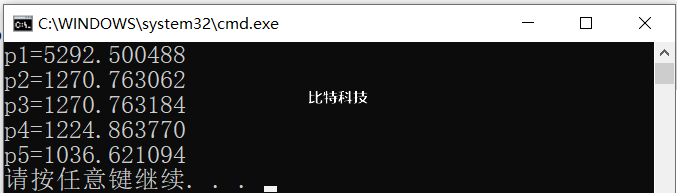3、购房从银行贷了一笔款d,准备每月还款额为p,月利率为r,计算多少月能还清。设d为300 000元,p为6000元,r为1%。对求得的月份取小数点后一位,对第2位按四舍五人处理。
提示:计算还清月数m的公式如下: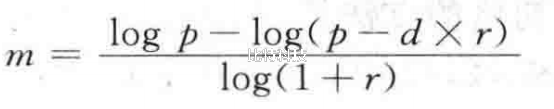可以将公式改写为: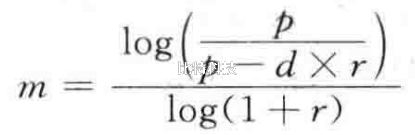C的库函数中有求对数的函数log10,是求以10为底的对数,log§表示log p。
题目解析:
该题的关键主要是利用数学函数库中的log函数进行求解，然后月份要求按照小数点后一位进行四舍五入进行处理，只需要在输出时进行格式控制即可。
代码示例:
#include<stdio.h>
#include <math.h>

int main()
{
float d = 300000, p = 6000, r = 0.01, m;
m = log10(p / (p - d*r)) / log10(1 + r);
printf("m = %3.1f\n", m);
return 0;
}

运行结果: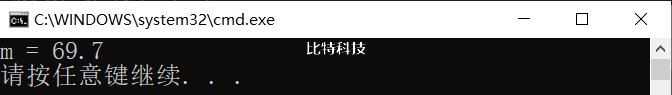4、分析下面的程序:
#include<stdio.h>
int main()
{
char c1, c2;
c1 = 97;
c2 = 98;
printf("c1=%c, c2=%c\n", c1, c2);
printf("c1=%d, c2=%d\n", c1, c2);
return 0;
}

(1)运行时会输出什么信息?为什么?
​     答：会输出 ：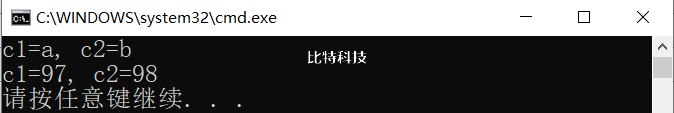​                          c1=97, c2=98
​            因为第一行十一字符形式输出，所以会吧97和98当作相依字符的ascii值，输出的时候按照字符显示，
​            第二行由于是以整形输出，所以输出对应整数值
(2)如果将程序第4,5行改为
c1 = 197;
c2 = 198;
运行时会输出什么信息?为什么?
​    答: 会输出：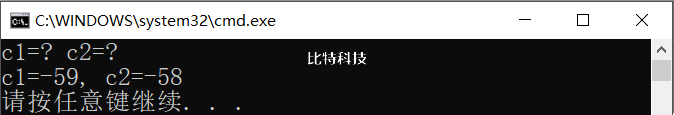​          因为c1和c2为char类型，其保存的数据范围为-128~127，当c1=197,c2=198时，已经超出了char类型所能够保存的数据范围，此时会进行相应的转换为c1=-59,c2=-58，只是第一行以字符输出时，c1=-59,c2=-58为不可显示字符，所以输出了？,而第二行以整数输出时就进行了相应的整数显示。
(3)如果将程序第3行改为
int c1, c2;
运行时会输出什么信息?为什么?
​    答: 会输出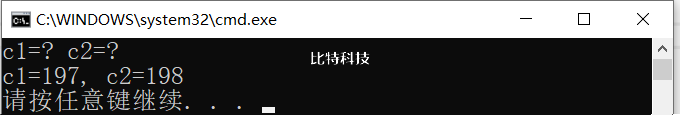​         因为c1和c2为int时，第二行以整形输出自然能够保存的下，但第一行以字符输出时，虽然数据int能够储存，但是在输出时需要准换为字符，转换后数据就超出了范围，此时c1=-59,c2=-58，两者都为不可显示字符，所以就显示了？。
5、用下面的scanf 函数输人数据,使a=3,b=7,x=8.5,y=71. 82,cl=‘A’,c2=‘a’。在键盘上应如何输入?
#include<stdio.h>
int main()
{
int a, b;
float x, y;
char c1, c2;
scanf("a=%db=%d", &a, &b);
scanf("%f%e",&x, &y);
scanf("%c%c",&c1, &c2);
return 0;
}

题目解析:
此题主要考察格式化输入，整形输入a和b时，必须按照a=3b=7的格式输入，浮点数输入时，%e代表科学记数法输入，字符输入c1和c2时，中间不能有任何空格
运行结果: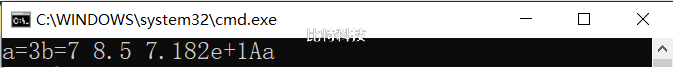6、请编程序将“China"译成密码，密码规律是:用原来的字母后面第4个字母代替原来的字母。例如,字母“A”后面第4个字母是“E”,用“E”代替“A”。因此,“China"应译为“Glmre”。请编一程序,用赋初值的方法使cl,c2,c3,c4,c5这5个变量的值分别为’C’,‘h’,‘i’,‘n’,‘a’ ,经过运算,使c1,c2,c3,c4,c5 分别变为’G’,‘l’,‘m’,‘r’,‘e’。分别用putchar函数和printf函数输出这5个字符。
题目解析:
密码转换的关键在于相应字符的ascii加4进行赋值修改原来的字符即可，修改完成后即为相应的密码，在使用putchar和printf进行相应输出即可。
代码示例:
#include<stdio.h>

int main()
{
char c1 = 'C', c2 = 'h', c3 = 'i', c4 = 'n', c5 = 'a';
c1 = c1 + 4;
c2 = c2 + 4;
c3 = c3 + 4;
c4 = c4 + 4;
c5 = c5 + 4;

//使用putchar输出
printf("使用putchar输出: ");
putchar(c1);
putchar(c2);
putchar(c3);
putchar(c4);
putchar(c5);
printf("\n");

//使用printf输出
printf("使用putchar输出: %c%c%c%c%c\n", c1, c2, c3, c4, c5);
return 0;
}

运行结果: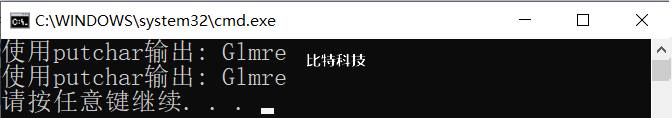7、设圆半径r=1.5,圆柱高h=3,求圆周长、圆面积、圆球表面积、圆球体积、圆柱体积。用scanf输人数据，输出计算结果，输出时要求有文字说明,取小数点后2位数字。请编程序。
题目解析:
此题主要理解圆柱的各种求法，其次要求取小数点后两位，只需在打印时进行格式控制即可。
代码示例:
#include<stdio.h>

int main()
{
float h, r, l, s, sq, vq, vz;
float pi = 3.141526;
printf("请输入圆半径r，圆柱高h∶");
scanf("%f %f", &r, &h);               //要求输入圆半径r和圆柱高h
l = 2 * pi*r;                          //计算圆周长l
s = r*r*pi;                          //计算圆面积s
sq = 4 * pi*r*r;                       //计算圆球表面积sq
vq = 3.0 / 4.0*pi*r*r*r;               //计算圆球体积vq
vz = pi*r*r*h;                       //计算圆柱体积vz
printf("圆周长为:       l=%6.2f\n", l);
printf("圆面积为:       s=%6.2f\n", s);
printf("圆球表面积为:   sq=%6.2f\n", sq);
printf("圆球体积为:     v=%6.2f\n", vq);
printf("圆柱体积为:     vz=%6.2f\n", vz);
return 0;
}


运行结果: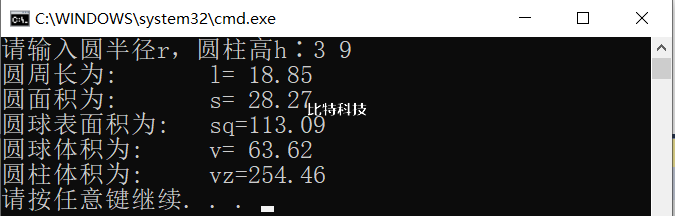8.编程序,用getchar函数读人两个字符给c1和c2，然后分别用putchar函数和printf函数输出这两个字符。思考以下问题:
(1)变量cl和c2应定义为字符型、整型还是二者皆可?
(2)要求输出cl和c2值的ASCII码,应如何处理?用putchar函数还是printf函数?
(3)整型变量与字符变量是否在任何情况下都可以互相代替?如:
char c1,c2;
与
int cl,c2;
是否无条件地等价?
题目解析:
(1)变量cl和c2应定义为字符型、整型还是二者皆可?
​    答：c1和c2 应定义为整形，因为定义为字符有可能返回的整数会超出字符的存储范围
(2)要求输出cl和c2值的ASCII码,应如何处理?用putchar函数还是printf函数?
​    答：需要使用printf函数，打印是以整形形式打印
(3)整型变量与字符变量是否在任何情况下都可以互相代替
​     答：不是，因为两者的存储范围不同，如果整数超出了字符的存储范围，则不能相互替换
代码示例:
int main()
{
int c1, c2;
printf("请输入两个字符c1,c2:");
c1 = getchar();
c2 = getchar();
printf("用putchar语句输出结果为:");
putchar(c1);
putchar(c2);
printf("\n");
printf("用printf语句输出结果为:");
printf("%c %c\n", c1, c2);
return 0;
}

运行结果: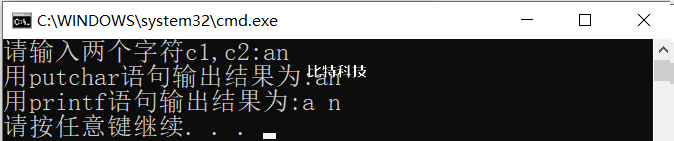c语言程序设计第五版谭浩强课后答案更多：
c程序设计第五版谭浩强习题答案 第二章课后答案


展开全文• 二章：算法----程序的灵魂 1. 什么是算法？试从日常生活中找3个例子，描述它们的算法 算法：简而言之就是求解问题的步骤，对特定问题求解步骤的一种描述。 比如生活中的例子： 考大学 首先填报志愿表、交报名费...
• C程序设计第五版 谭浩强 清华大学出版社 课后答案 习题解析 微信扫描小程序二维码查看答案 第一章 程序设计和C语言 第二章 算法—程序的灵魂 第三章 最简单的C程序设计—顺序程序设计 第四章 选择结构程序设计 第...C语言
• C程序设计谭浩强第五版课后答案 第四章习题答案 scanf出问题请看：超简单的scanf错误修改 4. 有3个整数a, b, c，由键盘输入，输出其中最大的数。 #define _CRT_SECURE_NO_WARNINGS #include <stdio.h> int ...c语言
• c程序设计第五版谭浩强习题答案 第四章 选择结构程序设计 1. 什么是算术运算？什么是关系运算？什么是逻辑运算？ 【答案解析】 算熟运算： 算术运算即“四则运算”，是加法、减法、乘法、除法、乘方、开方等几种运算...
• c程序设计第五版课后答案谭浩强 1.请画出例5.6中给出的3个程序段的流程图 流程图1： 流程图2： 流程图3： 2.请补充例5. 7程序,分别统计当“fabs(t)>= le- 6”和“fabs(t)> = le- 8”时执行循环体的次数。 ...c程序设计
• 3章 最简单的C程序设计——顺序程序设计 4章 选择结构程序设计 5章 循环结构程序设计 6章 利用数组处理批量数据 7章 用函数实现模块化程序设计 8章 善于利用指针 9章 用户自己建立数据类型 10...
• 谭浩强第五版C程序设计的课后习题答案--（第五章，拍不过来，后边的后续每天上传一章节），我无法扫描文件，所以我拍了高清图片，我也是用的这个，怕制作成pdf不高清，所以原图上传了，需要的下载。C语言
• 谭浩强C程序设计第版第五章课后答案 2.测试一下一个是5000001次一个是50000001次 3.最大公约数用辗转相除法，最大公约数与最小公倍数的乘积正好是两个数的乘积 #include <stdio.h> int main() { int m,n; ......